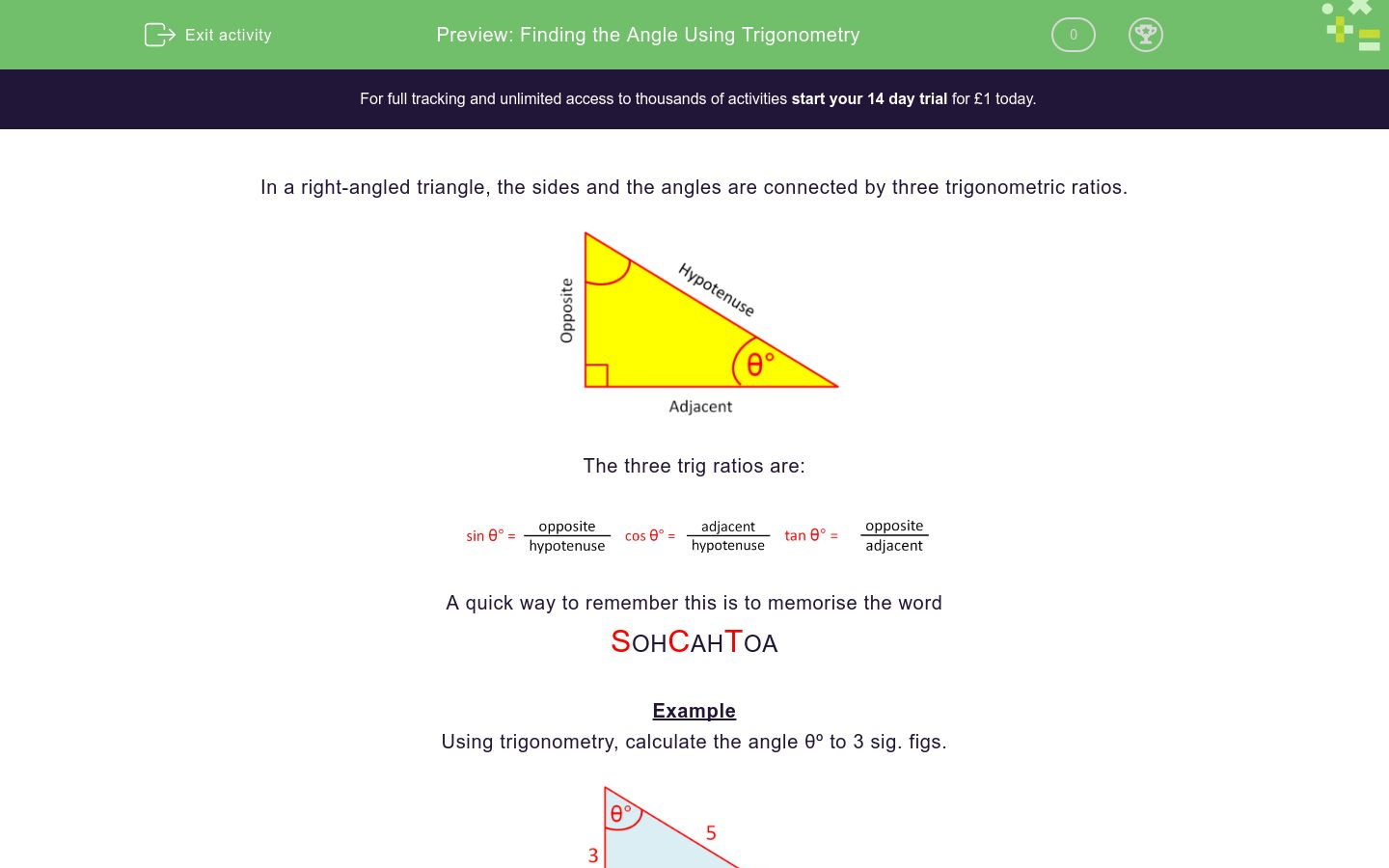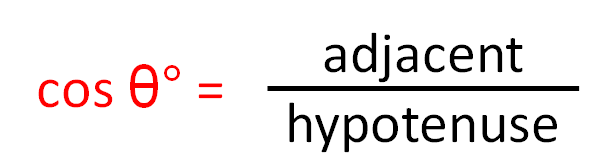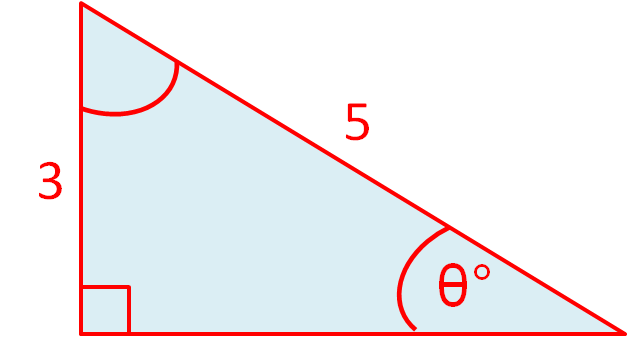# Finding the Angle Using Trigonometry

In this worksheet, students use trigonometry to calculate the angle in right-angled triangles.Key stage:  KS 3

Curriculum topic:   Geometry and Measures

Curriculum subtopic:   Solve Right-Angled Triangle Problems

Difficulty level:### QUESTION 1 of 10

In a right-angled triangle, the sides and the angles are connected by three trigonometric ratios.The three trig ratios are:A quick way to remember this is to memorise the word

SOHCAHTOA

Example

Using trigonometry, calculate the angle θº to 3 sig. figs.Label the sides adjacent, opposite and hypotenuse in relation to the angle θº.

The given sides are the adjacent and the hypotenuse.SOHCAHTOA

The correct trig ratio is COS.

Set up the trig equation to get:

cos θ = 3/5 = 3 ÷ 5 = 0.6

θ = cos-1 (0.6)

θ = 53.1º (3 s.f.)

Using trigonometry, calculate the angle θº to 3 sig. figs.

(just write the number)Using trigonometry, calculate the angle θº to 3 sig. figs.

(just write the number)Using trigonometry, calculate the angle θº to 3 sig. figs.

(just write the number)Using trigonometry, calculate the angle θº to 3 sig. figs.

(just write the number)Using trigonometry, calculate the angle θº to 3 sig. figs.

(just write the number)Using trigonometry, calculate the angle θº to 3 sig. figs.

(just write the number)Using trigonometry, calculate the angle θº to 3 sig. figs.

(just write the number)Using trigonometry, calculate the angle θº to 3 sig. figs.

(just write the number)Using trigonometry, calculate the angle θº to 3 sig. figs.

(just write the number)Using trigonometry, calculate the angle θº to 3 sig. figs.

(just write the number)• Question 1

Using trigonometry, calculate the angle θº to 3 sig. figs.

(just write the number)36.9
EDDIE SAYS
θ = sin-1(3/5)
• Question 2

Using trigonometry, calculate the angle θº to 3 sig. figs.

(just write the number)36.9
EDDIE SAYS
θ = tan-1(3/4)
• Question 3

Using trigonometry, calculate the angle θº to 3 sig. figs.

(just write the number)53.1
EDDIE SAYS
θ = tan-1(4/3)
• Question 4

Using trigonometry, calculate the angle θº to 3 sig. figs.

(just write the number)26.4
EDDIE SAYS
θ = sin-1(4/9)
• Question 5

Using trigonometry, calculate the angle θº to 3 sig. figs.

(just write the number)38.9
EDDIE SAYS
θ = cos-1(7/9)
• Question 6

Using trigonometry, calculate the angle θº to 3 sig. figs.

(just write the number)51.3
EDDIE SAYS
θ = tan-1(5/4)
• Question 7

Using trigonometry, calculate the angle θº to 3 sig. figs.

(just write the number)45.6
EDDIE SAYS
θ = sin-1(5/7)
• Question 8

Using trigonometry, calculate the angle θº to 3 sig. figs.

(just write the number)38.1
EDDIE SAYS
θ = tan-1(4/5.1)
• Question 9

Using trigonometry, calculate the angle θº to 3 sig. figs.

(just write the number)41.8
EDDIE SAYS
θ = sin-1(6/9)
• Question 10

Using trigonometry, calculate the angle θº to 3 sig. figs.

(just write the number)55.5
EDDIE SAYS
θ = cos-1(5.1/9)
---- OR ----

Sign up for a £1 trial so you can track and measure your child's progress on this activity.

### What is EdPlace?

We're your National Curriculum aligned online education content provider helping each child succeed in English, maths and science from year 1 to GCSE. With an EdPlace account you’ll be able to track and measure progress, helping each child achieve their best. We build confidence and attainment by personalising each child’s learning at a level that suits them.

Get started